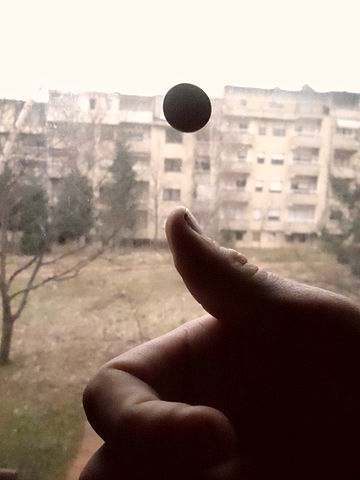# What a game!Two players, Nihar and I, are playing a game in which we alternate tossing a fair coin and the first player to get a head wins. Given that I toss first, the probability that Nihar wins the game is $\dfrac{\alpha}{\beta}$, where $\alpha$ and $\beta$ are coprime positive integers.

Find $\alpha + \beta$.

×